## Page Fault in OS-

Before you go through this article, make sure that you have gone through the previous article on Page Fault in OS.

We have discussed-

• When a page fault occurs, the required page has to be brought from the secondary memory.
• If all the frames of main memory are already occupied, then a page has to be replaced.
• Page Replacement Algorithms help to decide which page should be replaced.

## Effect of Increasing Number of Frames-

• The number of page faults should either decrease or remain constant on increasing the number of frames in main memory.
• But sometimes the unusual behavior is observed.
• Sometimes, on increasing the number of frames in main memory, the number of page faults also increase.

Belady’s Anomaly is the phenomenon of increasing the number of page faults on increasing the number of frames in main memory.

Following page replacement algorithms suffer from Belady’s Anomaly-

• FIFO Page Replacement Algorithm
• Random Page Replacement Algorithm
• Second Chance Algorithm

## NOTE-

In the above discussion,

• “Algorithms suffer from Belady’s Anomaly” does not mean that always the number of page faults will increase on increasing the number of frames in main memory.
• This unusual behavior is observed only sometimes.

• An algorithm suffers from Belady’s Anomaly if and only if it does not follow stack property.
• Algorithms that follow stack property are called as stack based algorithms.
• Stack based algorithms do not suffer from Belady’s Anomaly.
• This is because these algorithms assign priority to a page for replacement that is independent of the number of frames in the main memory.

## Examples-

Following page replacement algorithms are stack based algorithms-

1. LRU Page Replacement Algorithm
2. Optimal Page Replacement Algorithm

Hence, they do not suffer from Belady’s Anomaly.

## Stack Property-

Consider-

• Initially, we had ‘m’ number of frames in the main memory.
• Now, the number of frames in the main memory is increased to ‘m+1’.

According to stack property-

• At each stage, the set of pages that were present in the main memory when number of frames is ‘m’ will be compulsorily present in the corresponding stages in main memory when the number of frames is increased to ‘m+1’.

The following illustrations explain it clearly.

## Illustration-01: For Optimal Page Replacement Algorithm-

Consider the reference string is-

0, 1, 2, 3, 0, 1, 4, 0, 1, 2, 3, 4

Now, observe the following two cases-

### Case-01: When frame size = 3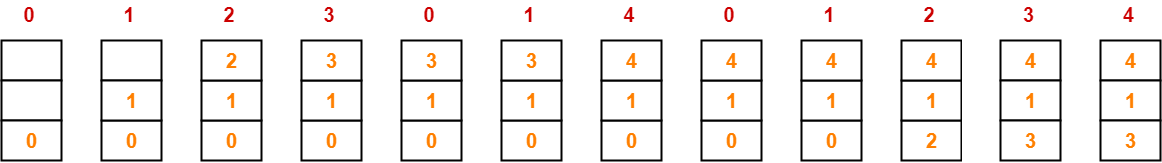Number of page faults = 7

### Case-02: When frame size = 4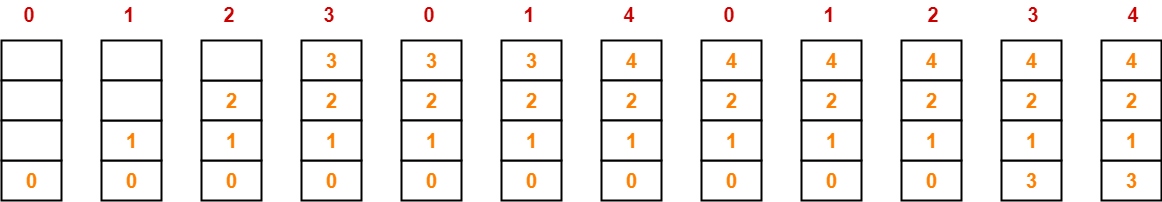Number of page faults = 6

From here, we can observe-

• At all the stages in case-02, main memory compulsorily contains the set of pages that are present in the corresponding stages in case-01.
• Thus, optimal page replacement algorithm follows the stack property.
• Hence, it does not suffer from Belady’s Anomaly.
• As a proof, number of page faults decrease when the number of frames is increased from 3 to 4.

## Illustration-02: For LRU Page Replacement Algorithm-

Consider the reference string is-

0, 1, 2, 3, 0, 1, 4, 0, 1, 2, 3, 4

Now, observe the following two cases-

### Case-01: When frame size = 3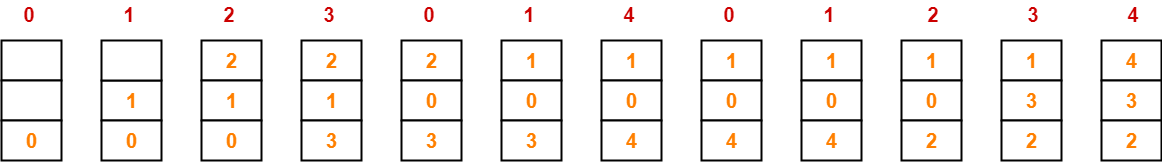Number of page faults = 10

### Case-02: When frame size = 4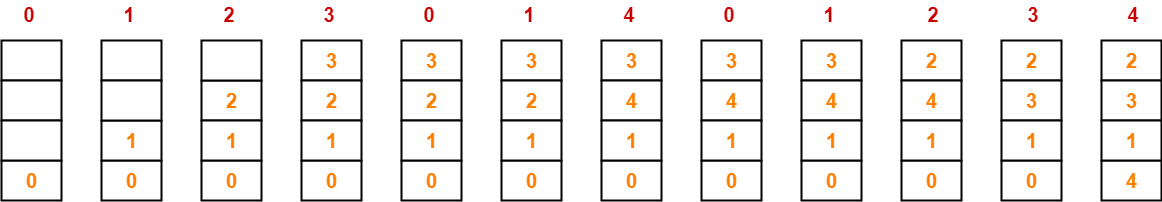Number of page faults = 8

From here, we can observe-

• At all the stages in case-02, main memory compulsorily contains the set of pages that are present in the corresponding stages in case-01.
• Thus, LRU page replacement algorithm follows the stack property.
• Hence, it does not suffer from Belady’s Anomaly.
• As a proof, number of page faults decrease when the number of frames is increased from 3 to 4.

## Illustration-03: For FIFO Page Replacement Algorithm-

Consider the reference string is-

0, 1, 2, 3, 0, 1, 4, 0, 1, 2, 3, 4

Now, observe the following two cases-

### Case-01: When frame size = 3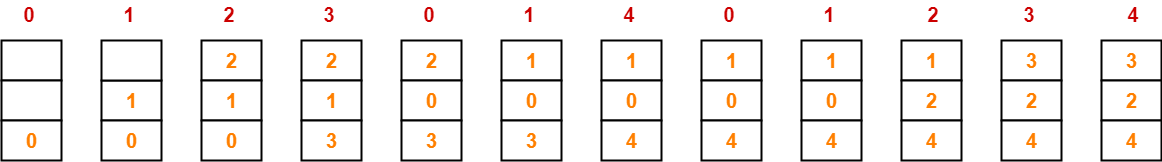Number of page faults = 9

### Case-02: When frame size = 4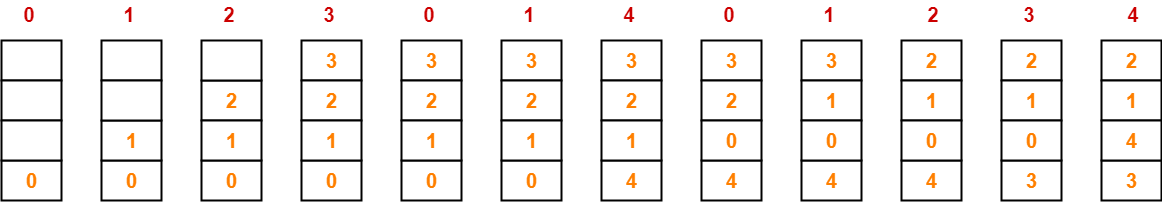Number of page faults = 10

From here, we can observe-

• At stage-07 and stage-08 in case-02, main memory does not contain the set of pages that are present in the corresponding stages in case-01.
• Thus, FIFO page replacement algorithm does not follow the stack property.
• Hence, it suffers from Belady’s Anomaly.
• As a proof, number of page faults increase when the number of frames is increased from 3 to 4.

## NOTE-

In the above illustration,

• FIFO Page Replacement Algorithm suffers from Belady’s Anomaly.
• It would be wrong to say that “FIFO Page Replacement Algorithm always suffer from Belady’s Anomaly”.